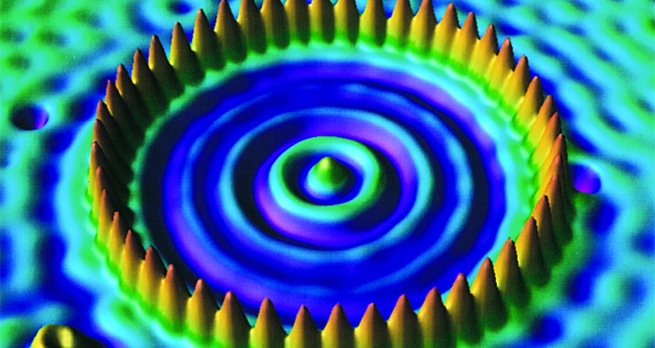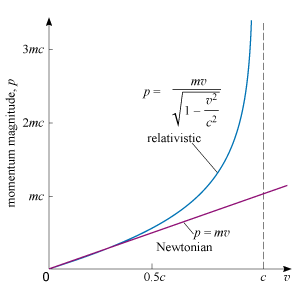Collisions and conservation laws

Start this free course now. Just create an account and sign in. Enrol and complete the course for a free statement of participation or digital badge if available.

Free course

# 7.1 Relativistic momentum

According to Einstein’s theory the relativistic momentum of a particle with mass and velocity is given by

Equation label: (12)

where is the speed of the particle and is the speed of light in a vacuum. The speed of light in a vacuum, , plays an important role throughout special relativity. Among other things it represents an upper limit to the speed of any particle.

Equation 12 implies that the momentum of a particle increases more rapidly with increasing speed than the Newtonian relation () predicts. This is shown in Figure 6, where the behaviour of the Newtonian and relativistic definitions of momentum magnitude are compared. You can see the good agreement at low speed, but you can also see the increasing discrepancy as the speed increases. Note that the relativistic definition does not extend beyond . This reflects the fact that in special relativity it is impossible to accelerate a particle with mass to the speed of light, as you will soon see.Figure 6 The magnitude of the momentum of a particle of mass plotted against the particle’s speed according to Newtonian mechanics and special relativity. The Newtonian relation closely approximates that of relativity for values of that are small compared with the speed of light, .
S217_1